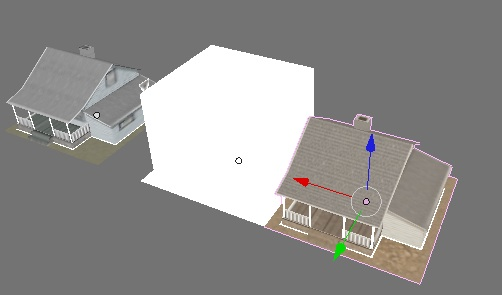# A Pythonic “Center New” in Blender

#### Wed 28 April 2010, tagged: GameDev Python Blender

Part of my Art pipeline for Tentacles is to import a directory of 3DS files. Each of these files is a map piece and the map pieces are instanced to create a map. That way I can store the geometry for a shack once but instance it across the level many times with a unique transform (scale, translation and rotation).Here you can see two map pieces and the big white box is the collision mesh that will be used for the map pieces

Upon importing the 3DS file I join the separate meshes together into one mesh and then reset the center of the model in the XY (ground) plane so that the map pieces can be snapped together and tiled easily.

## Center New

To move the center of an object to the center of the mesh Blender has a button calledCenter New (Editing Pane, Mesh Subpanel). Unfortunately there isn’t an easy way to invoke this from within python.

I found this code on the internet quite quickly but I found that for some models it just wasn’t working. Infact for some models if I called the function twice the center changed twice!

I rejigged the code a little to get it to work every time on Blender 2.49. Other then renaming the variables to make it a little easier to read I get the objects bounding box before manipulating the object and it’s meshes matrix. Use

 ``` 1 2 3 4 5 6 7 8 9 10 11 12 13 14 15 16 17 18 19 20 21 22 23 24 25 26 27 28 29``` ``````def center_new(bobject, xfactor=1, yfactor=1, zfactor=0): bbbox= bobject.getBoundBox() bmesh=bobject.getData(0, 1) matrix= bobject.getMatrix() bmesh.transform(matrix) bmesh.update() matrix=Blender.Mathutils.Matrix( [1.0,0.0,0.0,0.0], [0.0,1.0,0.0,0.0], [0.0,0.0,1.0,0.0], [0.0,0.0,0.0,1.0]) bobject.setMatrix(matrix) bboxcenter=[bbbox[n] + ( bbbox[-2][n] - bbbox[n] )/2.0 for n in [0,1,2] ] matrix= bobject.getMatrix() matrix=Blender.Mathutils.Matrix(matrix[:], matrix[:], matrix[:], [-bboxcenter * xfactor,-bboxcenter * yfactor, -bboxcenter * zfactor,1.0]) bmesh.transform(matrix) bmesh.update() matrix=Blender.Mathutils.Matrix(matrix[:], matrix[:], matrix[:], [bboxcenter * xfactor, bboxcenter * yfactor, bboxcenter * zfactor, 1.0]) bobject.setMatrix(matrix) ``````